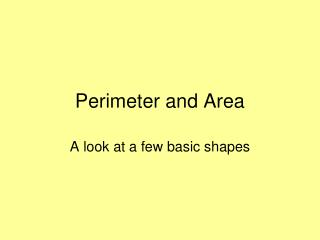Download PresentationPerimeter and Area

# Perimeter and Area - PowerPoint PPT PresentationDownload Presentation## Perimeter and Area

- - - - - - - - - - - - - - - - - - - - - - - - - - - E N D - - - - - - - - - - - - - - - - - - - - - - - - - - -
##### Presentation Transcript

1. Perimeter and Area A look at a few basic shapes

2. Perimeter

3. This little square represents a bigger square, one yard in length, and one yard in width.

4. And this is Stamford Bridge (football!)

5. 110 yards 75 yards and 75 yards wide

6. 110 yards 75 yards

7. 110 yards 110 yards 75 yards 75 yards What is the perimeter of the football pitch?

8. 110 yards 110 yards 75 yards 75 yards 110 + 75 + 110 + 75 = 370 yards

9. Our classroom is approximately 8 metres by 5 metres What is the perimeter?

10. 8 m Our classroom 5 m

11. 8 m 8 m Our classroom 5 m 5 m 8 + 5 + 8 + 5 = 26 metres

12. Question? How do we find the perimeter of a triangle? Answer: Let Google Maps do the measuring for us.

13. Or 2922 miles

14. 3 m 5 m 4 m The perimeter of this triangle? 3 + 4 + 5 = 12 metres

15. 13 cm 5 cm 12 cm The perimeter of this triangle? 5 + 12 + 13 = 30 cm

16. These TETROMINOES are all made from four squares Do they all have the same perimeter?

17. I get the following: 10 units 10 units 10 units 8 units 10 units

18. Pause for Play Draw some shapes with a perimeter of 20 centimetres.

19. Let’s go back to Stamford Bridge What is the perimeter of that circle in the middle? Actually, on a circle it is called the circumference

20. 10 yards According to BBC Sport, it has a radius of 10 yards. Which is from the centre of the circle to the circumference.

21. 20 yards 10 yards If the radius is 10 yards, then the diameter is 20 yards. The circumference is about three times the diameter. So the circumference is about 3 x 20 = 60 yards

22. 20 yards 60 yards

23. 20 yards 10 yards If you have a calculator, then you could say it is 3.1, or 3.14, or 3.142 times the diameter. If you have a posh calculator, you could use the πbutton.

24. Pi – the Greek letter π, which represents the ratio of the circumference to the diameter of a circle. • We can’t actually write it down exactly. • But we can write it to as many decimal places as we want.

25. 3.14159265358979323846264338327950288419716939937510582097494459230781640628620899862803482534211706798214808651328230664709384460955058223172535940812848111745028410270193852110555964462294895493038196442881097566593344612847564823378678316527120190914564856692346034861045432664821339360726024914127372458700660631558817488152092096282925409171536436789259036001133053054882046652138414695194151160943305727036575959195309218611738193261179310511854807446237996274956735188575272489122793818301194912983367336244065664308602139494639522473719070217986094370277053921717629317675238467481846766940513200056812714526356082778577134275778960917363717872146844090122495343014654958537105079227968925892354201995611212902196086403441815981362977477130996051870721134999999837297804995105973173281609631859502445945534690830264252230825334468503526193118817101000313783875288658753320838142061717766914730359825349042875546873115956286388235378759375195778185778053217122680661300192787661119590921642019…3.14159265358979323846264338327950288419716939937510582097494459230781640628620899862803482534211706798214808651328230664709384460955058223172535940812848111745028410270193852110555964462294895493038196442881097566593344612847564823378678316527120190914564856692346034861045432664821339360726024914127372458700660631558817488152092096282925409171536436789259036001133053054882046652138414695194151160943305727036575959195309218611738193261179310511854807446237996274956735188575272489122793818301194912983367336244065664308602139494639522473719070217986094370277053921717629317675238467481846766940513200056812714526356082778577134275778960917363717872146844090122495343014654958537105079227968925892354201995611212902196086403441815981362977477130996051870721134999999837297804995105973173281609631859502445945534690830264252230825334468503526193118817101000313783875288658753320838142061717766914730359825349042875546873115956286388235378759375195778185778053217122680661300192787661119590921642019…

26. Pause for Play 6 cm 4 cm Which has the greatest perimeter? 8 cm 8 cm

28. 5 m 8 m What do we use to measure area?

29. Square metres (or square yards, or square inches, or square centimetres…)

30. 8 m How many square metres? 5 m

31. 8 m2

32. 8 m 5 m 5 x 8 = 40 square metres, or 40 m2

33. Pause for Play Draw some rectangles with an area of 20 square centimetres. Do they have the same perimeter?

34. 110 yards 75 yards What, in square yards, is the area of Stamford Bridge?

35. 110 yards 75 yards 110 x 75 = 8250 square yards

36. What about a triangle -how do we find the area?

38. What if we ‘double up’? 5 cm 12 cm

39. 5 cm 12 cm Area of the rectangle? Area of the triangle?

40. 5 cm 12 cm Area of the rectangle = 60 cm2 Area of the triangle = 30 cm2

41. 3 cm 8 cm Slightly more complicated:

42. But we can still ‘double up’

43. 8 cm But we can still ‘double up’ 3 cm

44. 3 cm 8 cm Area of rectangle = 3 x 8 = 24 Area of triangle = (3 x 8) ÷ 2 = 12 cm2